# Numpy log explained

In this tutorial, you’ll learn how to use the Numpy log function to calculate logarithms in Python. This tutorial will explain the syntax of np.log, and it will also show you step-by-step examples of Numpy log that you can run yourself.

If you have something specific that you need, you can click on any of the following links:

Those links will take you to specific parts of the tutorial, so you can get what you need quickly.

However, you’ll definitely learn more if you read the whole tutorial, especially if you’re new to Numpy.

That being said, let’s do a quick review of Numpy and what it is.

## A quick review of Numpy

Let’s quickly review Numpy.

Numpy is a package for the Python programing language for working with numerical data.

Specifically, Numpy enables you to create and perform operations on a Python object called a Numpy array.

A Numpy array is essentially a structure that contains numeric data in a row-and-column format.

To be clear, arrays can come in a variety of shapes and sizes, including 1-dimentional, 2-dimensional, and N-dimentional.

1-dimentional arrays, look something like this:And here’s an example of a 2-dimentional array:These are just examples though. Again, Numpy arrays can have a variety of shapes and sizes.

### Numpy has tools for creating Numpy arrays

Numpy has a variety of tools for creating Numpy arrays.

For example, there are tools to:

Those are just a few examples. There are quite a few more ways to create arrays.

### Numpy has tools to perform numeric computations

Once you have a Numpy array, you can also use Numpy to perform a variety of calculations on your array.

For example, you can use the Numpy exponential function to compute the exponential of the values in an array. Or you can use the Numpy power function to raise every value in an array to a specific power.

Similarly, you can compute the logarithm of every value in a Numpy array.

That’s exactly what we do with Numpy log.

## A quick introduction to Numpy log

The Numpy log function is fairly simple: we use it to compute the natural logarithm of the values in a Numpy array.

You’ll probably remember logarithms from mathematics classes.

The natural logarithm is the inverse of the exponential function,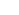, such that: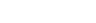Computing the log is fairly common in scientific tasks, and Numpy log gives us an easy way to compute the natural logarithm in Python.

Now that we’ve reviewed the basics, let’s take a look at the syntax.

## The syntax of np.log

The syntax of Numpy log is fairly simple.

Before we get into the details of the syntax though, I need to remind you of one syntactical convention that we’ll use.

#### A quick note about Numpy syntax

Typically, when we use Numpy functions, we call them with the prefix ‘`np`‘. This is a common convention among almost all Python coders who use Numpy.

To be able to use that prefix, you need to import Numpy with very specific syntax:

```import numpy as np
```

When we import Numpy with the code `import numpy as np`, it enables us to call Numpy functions starting with the alias, `np`.

For example, if you import Numpy this way, you can call the Numpy log function as `np.log()`.

Alternatively, if you import Numpy differently, you’ll have to call the function differently. For example, if you simply use the code `import numpy`, you would call the function as `numpy.log()`.

My point here is that exactly how you call the function depends on how you import Numpy.

Having said that, we’re going to stick with the convention of importing Numpy with ‘`import numpy as np`‘, and we’ll call the function as `np.log`.

### The syntax of np.log

Ok. Let’s take a look at the syntax.

The syntax of Numpy log is fairly simple.

Assuming that you’ve imported Numpy with the alias `np` (as described above), you’ll call the function as `np.log()`.Inside of the function, you provide a Numpy array of elements (or an “array-like” object). Numpy log will compute the logarithm for those elements.That’s the high-level view of the syntax, but there are some details that we should cover.

That being said, let’s quickly discuss the parameters of np.log.

### The parameters of np.log

The np.log function is simple, in that it only has a few parameters. (Many other Numpy functions have a large number of parameters, so np.log is relatively simple.)

The three parameters of np.log are `x`, `out`, and `where`.

Two of those parameters, the `out` parameter and the `where` parameter, are less commonly used, so we’re not going to cover them in this tutorial.

That being the case, let’s take a look at the `x` parameter and how it works.

###### `x` (required)

The `x` parameter enables you to provide an input to the function.

I’ll show you some examples of this in the examples section, but in a simple form, if you have an input array called `my_array`, you can pass that input to np.log as follows:

```np.log(my_array)
```

This parameter will accept inputs of a few different types. Numpy log accepts “array like” inputs, meaning that it accepts Numpy arrays, but also objects similar to Numpy arrays. For example, the `x` parameter will also accept a Python list as an input.

Keep in mind that you need to provide some input to the np.log function. You need to provide an input.

At the same time, although you need to provide an input to the `x` parameter, you don’t explicitly use the `x` parameter in your syntax.

Here’s what I mean.

In the above example, I mentioned that you would typically use the Numpy log function with syntax that looks like `np.log(my_array)`.

When you write your code like this, Numpy understands that `my_array` is being passed to the `x` parameter.

(If you don’t understand this, I recommend that you do some reading about positional arguments in Python.)

Ok. Now that we’ve discussed the syntax of np.log, let’s take a look at some examples.

## Examples of how to use Numpy log

Here, I’ll show you some step-by-step examples of how to use Numpy log.

We’ll start with an extremely simple example, and then increase the complexity from there.

Examples:

#### Run this code first

Before you run the examples, you’ll need to run the following code to import Numpy.

Remember, as I explained above, in order to call functions from Numpy, we need to import Numpy. And how exactly we import Numpy will impact the exact syntax for calling our function.

Having said that, the common convention is to import Numpy with the alias `np`. Almost all Python programmers who use Numpy use this convention.

So, you can import Numpy by running the following code.

```import numpy as np
```

Once you import Numpy, you’ll be ready to run the examples.

### EXAMPLE 1: Use np.log with a single number

First, we’ll start by running np.log on a single number.

Here, we’ll calculate the natural logarithm of the mathematical constant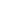, AKA, Euler’s number.

To do this, we’ll be able to use the constant `np.e` from Numpy.

If you print it out, you can see the value:

```print(np.e)
```

OUT:

```2.718281828459045
```

And now, let’s compute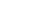with `np.log`.

```np.log(np.e)
```

OUT:

```1.0
```
##### Explanation

This is fairly simple.

Here, we’re computing the natural log of the constant. Because the function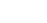is the inverse of the exponential,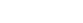.

If you’d like, try to run this code with some other numbers besides.

### EXAMPLE 2: Use np.log with a Python list

Now, we’ll run `np.log()` on a Python list.

We’ll run the code with the list of numbers from 1 to 4.

Let’s run the code:

```np.log([1,2,3,4])
```

OUT:

```array([0., 0.69314718, 1.09861229, 1.38629436])
```
##### Explanation

Here, `np.log` is just computing the natural log,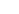, for every element of the list.Ultimately, the output is a Numpy array that contains the natural log of each element, `array([0., 0.69314718, 1.09861229, 1.38629436])`.

### EXAMPLE 3: Run Numpy log on a Numpy array

Now, we’ll use Numpy log on a Numpy array.

First, we’ll create an array of Numbers.

##### Create Numpy array

Here, we’re going to use the Numpy arange function to create an array of numbers from 1 to 4.

```my_array = np.arange(start = 1, stop = 5)
```

And let’s take a look at it:

```print(my_array)
```

OUT:

```[1 2 3 4]
```

The object `my_array` is technically a Numpy array, but it’s very similar to the list that we used in example 2. It contains the same numbers.

##### Use Numpy log

Now, we’ll use the Numpy log function on `my_array` to calculate the natural log of each number.

```np.log(my_array)
```

OUT:

```array([0., 0.69314718, 1.09861229, 1.38629436])
```
##### Explanation

This should be very easy to understand.

Here, we used np.log to calculate the natural logarithm,, of every element in the array.

The array contains the numbers `[1,2,3,4]`, so the output is `[ln(1), ln(2), ln(3), ln(4)]`.

These numbers calculate as `array([0., 0.69314718, 1.09861229, 1.38629436])`.

### EXAMPLE 4: Run Numpy log on a 2-dimensional Numpy array

Finally, let’s run one more example.

Here, we’ll run Numpy log on a 2-dimensional Numpy array.

###### Create 2D Numpy array

First, let’s just create our Numpy array. We’ll use np.arange to create a Numpy array with the values from 1 to 6, and we’ll reshape that array in to 2 dimensions using the Numpy reshape method.

```array_2d = np.arange(start = 1, stop = 7).reshape((2,3))
```

And let’s print it out, so you can see the contents.

```print(array_2d)
```

OUT:

```[[1 2 3]
[4 5 6]]
```

This is just a Numpy array with the values from 1 to 6, arranged in 2 rows and 3 columns.

###### Compute natural logarithm with Numpy

Now, let’s compute the natural logarithm using Numpy log.

```np.log(array_2d)
```

OUT:

```array([[0.        , 0.69314718, 1.09861229],
[1.38629436, 1.60943791, 1.79175947]])
```
###### Explanation

Again, np.log just computes the natural log,of every element in the input array.

In this case, the input was a 2 by 3 array (a 2-dimensional array with 2 rows and 3 columns), so the output has the same shape.

Do you still have questions about Numpy log?

The examples you’ve seen in this tutorial should be enough to get you started, but if you’re serious about learning Numpy, you should enroll in our premium course called Numpy Mastery.

There’s a lot more to learn about Numpy, and Numpy Mastery will teach you everything, including:

• How to create Numpy arrays
• How to use the Numpy random functions
• What the “Numpy random seed” function does
• How to reshape, split, and combine your Numpy arrays
• and more …

Moreover, it will help you completely master the syntax within a few weeks. You’ll discover how to become “fluent” in writing Numpy code.

Find out more here:

Joshua Ebner

Joshua Ebner is the founder, CEO, and Chief Data Scientist of Sharp Sight. Prior to founding the company, Josh worked as a Data Scientist at Apple. He has a degree in Physics from Cornell University.